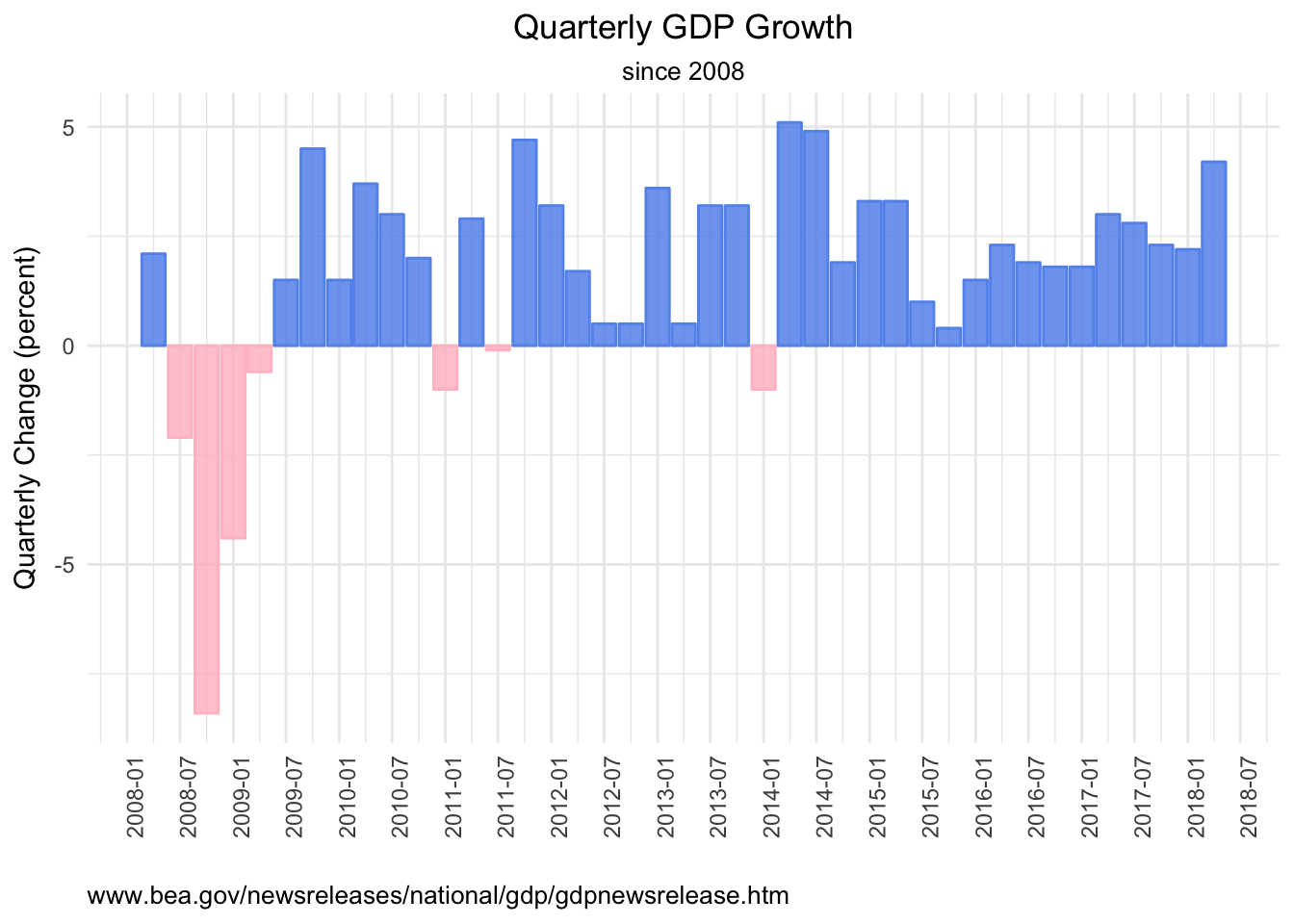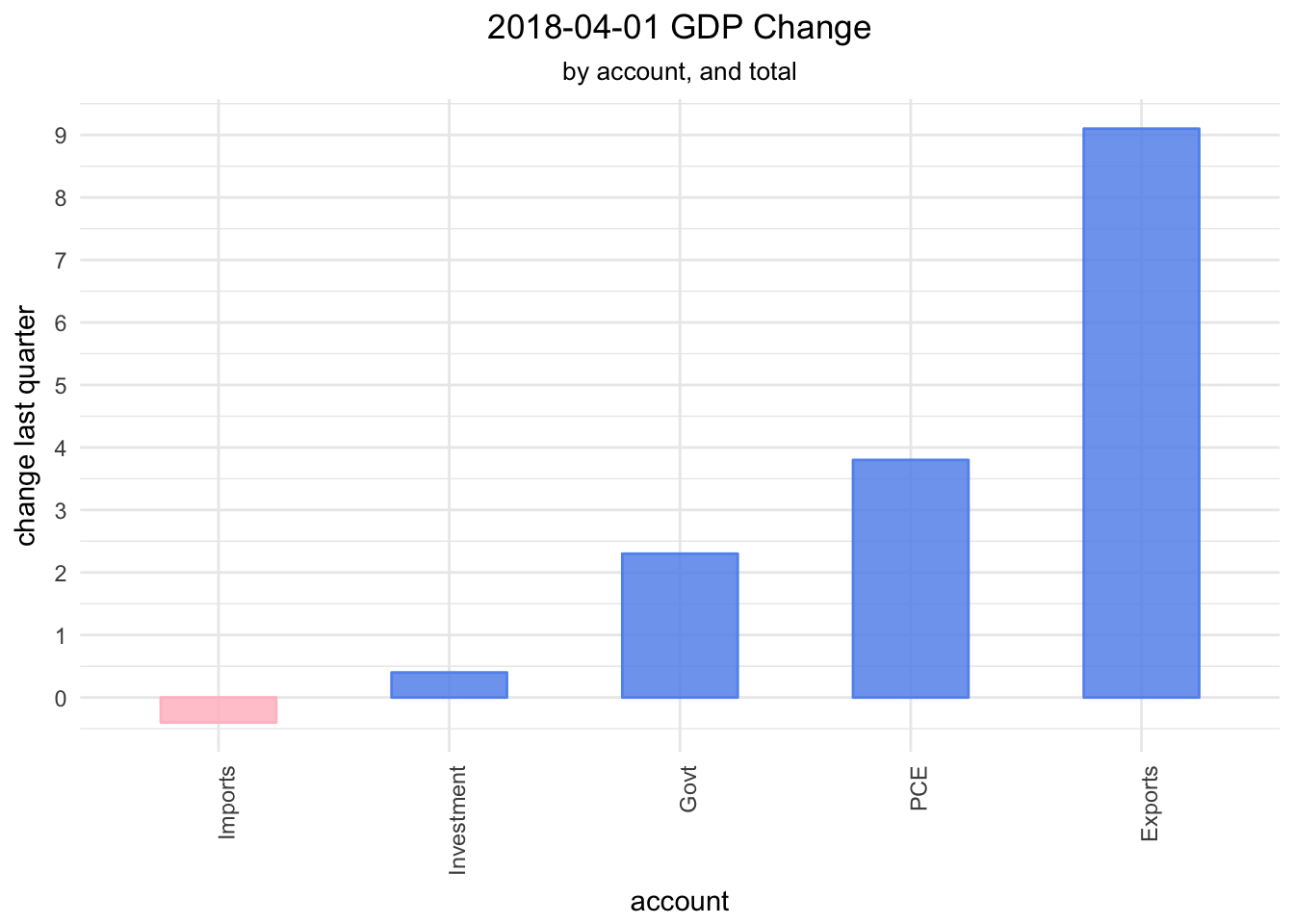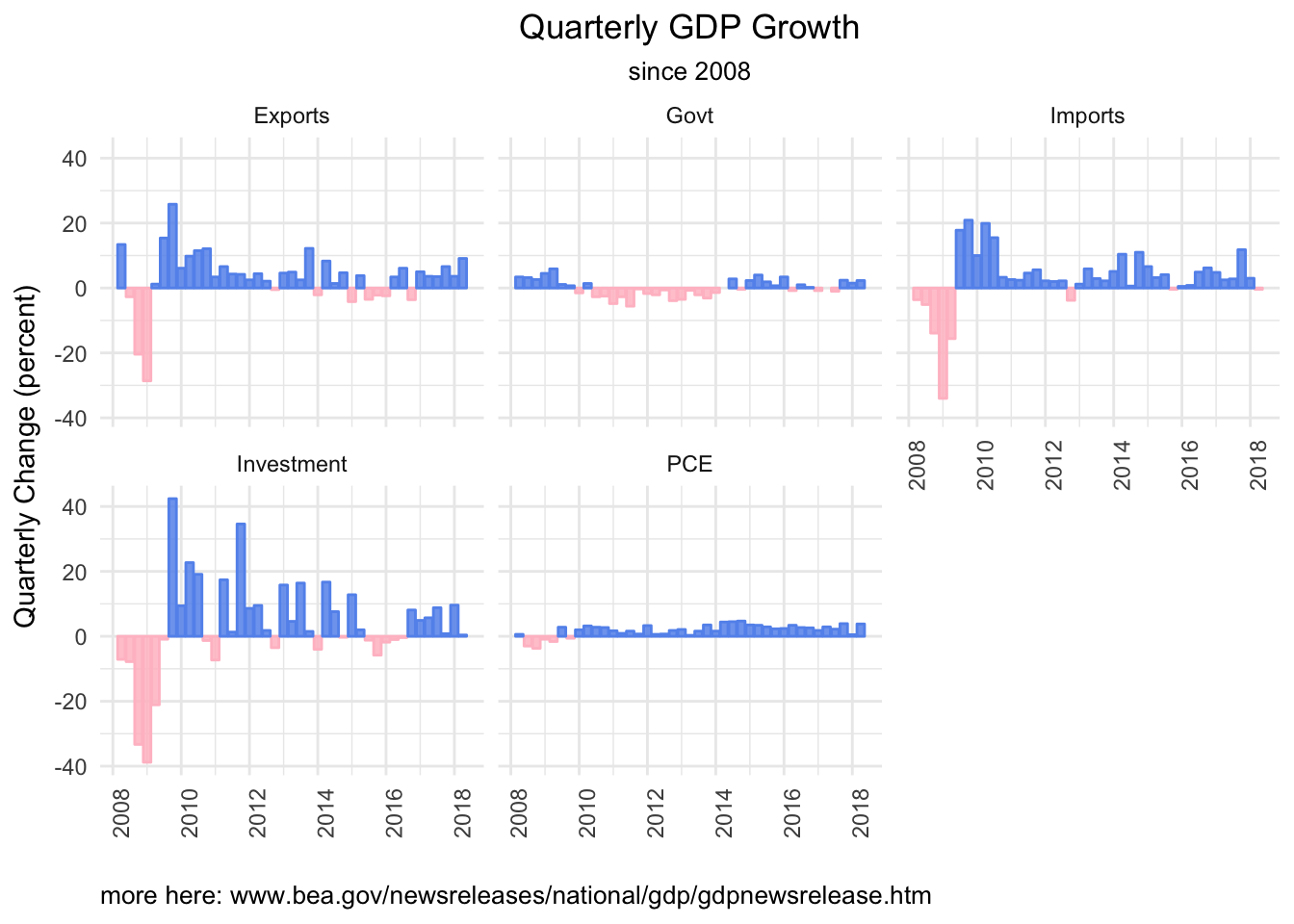# GDP Data via API

by Jonathan Regenstein

Today, we will look at the GDP data that is released every quarter or so by the Bureau of Economic Analysis (BEA), and get familiar with the BEA API (see the documentation here). For a primer on GDP in general, BEA publishes this guide.

To access the BEA API, we will need two packages, httr and jsonlite.

library(tidyverse)
library(tidyquant)
library(httr)
library(jsonlite)

We also need to know the API address and parameters to get. This information can be found in the API documentation referenced above. We supply our API key, the name of the data set (NIPA), the table name (T10101, which holds GDP data), and a frequency (Q, for quarterly).

"https://apps.bea.gov/api/data/?&UserID=Your-API-Key Here&method=GetData&DataSetName=NIPA&TableName=T10101&Frequency=Q&
Year=ALL&ResultFormat=JSON"

We will pass that URL to the fromJSON() function of the jsonlite package to access the API, and include simplifyDataFrame = FALSE and simplifyMatrix = FALSE to turn off some of the built-in data helpers.

key_string <-
paste("https://apps.bea.gov/api/data/?&UserID=",
my_api_key,
"&method=GetData&DataSetName=NIPA&TableName=T10101&Frequency=Q&Year=ALL&ResultFormat=JSON",
sep = "")

bea_gdp_api <-
fromJSON(key_string,
simplifyDataFrame = FALSE,
simplifyMatrix = FALSE)

str(bea_gdp_api, max=3)
List of 1
$BEAAPI:List of 2 ..$ Request:List of 1
.. ..$RequestParam:List of 8 ..$ Results:List of 5
.. ..$Statistic : chr "NIPA Table" .. ..$ UTCProductionTime: chr "2018-09-12T16:30:26.567"
.. ..$Dimensions :List of 9 .. ..$ Data             :List of 7125
.. ..$Notes :List of 1 That worked, but the returned data is a monster (remove that max = 3 argument and see what happens)! Let’s use pluck() from the purrr package to extract the data we want by calling pluck("BEAAPI","Results","Data"). That command plucks the list called BEAAPI, then the list called Results, then the list called Data. bea_gdp_api <- fromJSON("https://apps.bea.gov/api/data/?&UserID=084F6B76-36BE-431F-8F2A-54429DF5E04C&method=GetData&DataSetName=NIPA&TableName=T10101&Frequency=Q&Year=ALL&ResultFormat=JSON", simplifyDataFrame = FALSE, simplifyMatrix = FALSE) %>% pluck("BEAAPI","Results","Data") str(bea_gdp_api) List of 1$ :List of 9
..$TableName : chr "T10101" ..$ SeriesCode     : chr "A191RL"
..$LineNumber : chr "1" ..$ LineDescription: chr "Gross domestic product"
..$TimePeriod : chr "1962Q1" ..$ METRIC_NAME    : chr "Fisher Quantity Index"
..$CL_UNIT : chr "Percent change, annual rate" ..$ UNIT_MULT      : chr "0"
..$DataValue : chr "7.3" OK, we’re getting somewhere now - a list of 7125 lists of 9 elements. Looking at those 9 elements, we want the TimePeriod, the LineDescription, the DataValue, and for reasons we’ll see later, the SeriesCode. So, we want 4 items from each of those 7125 lists, and ideally we would like to convert them to a tibble. We can use map_df() from purrr to apply the extract() function from magrittr and select just the list elements we want, and convert the results to a tibble. By appending _df, we are telling map to return a data frame. bea_gdp_api <- fromJSON(key_string, simplifyDataFrame = FALSE, simplifyMatrix = FALSE) %>% pluck("BEAAPI","Results","Data") %>% map_df(magrittr::extract, c("LineDescription", "TimePeriod", "SeriesCode", "DataValue")) str(bea_gdp_api) Classes 'tbl_df', 'tbl' and 'data.frame': 7125 obs. of 4 variables:$ LineDescription: chr  "Gross domestic product" "Gross domestic product" "Gross domestic product" "Gross domestic product" ...
$TimePeriod : chr "1962Q1" "1962Q2" "1962Q3" "1962Q4" ...$ SeriesCode     : chr  "A191RL" "A191RL" "A191RL" "A191RL" ...
$DataValue : chr "7.3" "3.7" "5.0" "1.3" ... Much, much better. We now have a tibble, with 4 columns and 7125 rows. Let’s do some cleanup with rename() for better column names, and then make sure that the percent_change and quarter column are in a good format. bea_gdp_api <- bea_gdp_api %>% group_by(LineDescription) %>% rename(account = LineDescription, quarter = TimePeriod, percent_change = DataValue) %>% mutate(percent_change = as.numeric(percent_change), quarter = yq(quarter)) bea_gdp_api is now a tibble that holds the quarterly percentage change for each of the GDP accounts used by the BEA, including total GDP change. Let’s take a closer look at each of the accounts whose data we have. bea_gdp_api %>% count() # A tibble: 21 x 2 # Groups: account  account n <chr> <int> 1 Durable goods 285 2 Equipment 285 3 Exports 285 4 Federal 285 5 Fixed investment 285 6 Goods 855 7 Government consumption expenditures and gross investment 285 8 Gross domestic product 285 9 Gross domestic product, current dollars 285 10 Gross private domestic investment 285 # ... with 11 more rows We have 21 accounts or groups, including both Gross domestic product and Gross domestic product, current dollars. What are the other 19 groups? They are the sub-accounts that comprise GDP. Note that n = 855 for the Goods account and the Services account, but we have only 285 quarters of data. That’s because there are three accounts called Goods and Services (we’ll look at these three below). We can look at the Goods accounts by SeriesCode. bea_gdp_api %>% group_by(SeriesCode) %>% slice(1) %>% select(account, SeriesCode) %>% filter(account == "Goods") # A tibble: 3 x 2 # Groups: SeriesCode  account SeriesCode <chr> <chr> 1 Goods A253RL 2 Goods A255RL 3 Goods DGDSRL  This is why we grabbed the series codes, too. We need a way to figure out the true account for these three things labeled as Goods. A web search reveals that A253RL is for Real Exports of Goods (a third-level account), A255RL is for Real Imports of Goods (a third-level account), and DGDSRL is a second-level account and the Goods component of Real Personal Consumption and Expenditure (PCE). Let’s add better account/group names by with case_when(). bea_gdp_api %>% ungroup() %>% mutate(account = case_when(SeriesCode == "A253RL" ~ "Export Goods", SeriesCode == "A255RL" ~ "Import Goods", SeriesCode == "DGDSRL" ~ "Goods", TRUE ~ .$account)) %>%
group_by(account) %>%
count()
# A tibble: 23 x 2
# Groups:   account 
account                                                      n
<chr>                                                    <int>
1 Durable goods                                              285
2 Equipment                                                  285
3 Export Goods                                               285
4 Exports                                                    285
5 Federal                                                    285
6 Fixed investment                                           285
7 Goods                                                      285
8 Government consumption expenditures and gross investment   285
9 Gross domestic product                                     285
10 Gross domestic product, current dollars                    285
# ... with 13 more rows

We repeat that process for Services, which also had three accounts smooshed into one label.

bea_gdp_api %>%
group_by(SeriesCode) %>%
slice(1) %>%
select(account, SeriesCode) %>%
filter(account == "Services")
# A tibble: 3 x 2
# Groups:   SeriesCode 
account  SeriesCode
<chr>    <chr>
1 Services A646RL
2 Services A656RL
3 Services DSERRL    

Similar to with goods, DSERRL is the services component of PCE and is a second-level account. A656RL is imports of services (a third-level account), and A646RL is exports of services.

Let’s make our changes to both goods and services in the data. I’m also going to replace a few other accounts with shorter names, e.g., I will use “Govt” for “Government consumption expenditures and gross investment”.

bea_gdp_wrangled <-
bea_gdp_api %>%
ungroup() %>%
mutate(account = case_when(SeriesCode == "A253RL" ~ "Export Goods",
SeriesCode == "A255RL" ~ "Import Goods",
SeriesCode == "DGDSRL" ~ "Goods",
SeriesCode == "DSERRL" ~ "Services",
SeriesCode == "A656RL" ~ "Import Services",
SeriesCode == "A646RL" ~ "Export Services",
SeriesCode == "A822RL" ~ "Govt",
SeriesCode == "A006RL" ~ "Investment",
SeriesCode == "DPCERL" ~ "PCE",
TRUE ~ .$account)) %>% group_by(account) %>% select(-SeriesCode) bea_gdp_wrangled %>% count() # A tibble: 25 x 2 # Groups: account  account n <chr> <int> 1 Durable goods 285 2 Equipment 285 3 Export Goods 285 4 Export Services 285 5 Exports 285 6 Federal 285 7 Fixed investment 285 8 Goods 285 9 Govt 285 10 Gross domestic product 285 # ... with 15 more rows We now have 25 accounts, each with 285 observations. Let’s move to some visualization and check out how GDP has changed on a quarterly basis since 2008. bea_gdp_wrangled %>% filter(quarter > "2008-01-01") %>% filter(account == "Gross domestic product") %>% mutate(col_blue = if_else(percent_change > 0, percent_change, as.numeric(NA)), col_red = if_else(percent_change < 0, percent_change, as.numeric(NA))) %>% ggplot(aes(x = quarter)) + geom_col(aes(y = col_red), alpha = .85, fill = "pink", color = "pink") + geom_col(aes(y = col_blue), alpha = .85, fill = "cornflowerblue", color = "cornflowerblue") + ylab("Quarterly Change (percent)") + scale_x_date(breaks = scales::pretty_breaks(n = 20)) + labs(title = "Quarterly GDP Growth", subtitle = "since 2008", x = "", caption = "www.bea.gov/newsreleases/national/gdp/gdpnewsrelease.htm") + theme_minimal() + theme(axis.text.x = element_text(angle = 90, hjust = 1), plot.title = element_text(hjust = 0.5), plot.subtitle = element_text(hjust = 0.5), plot.caption=element_text(hjust=0))That’s a nice look at GDP quarterly change since 2008, but let’s go a bit deeper and visualize the four top-level components of GDP, which are personal consumption, net exports (or imports and exports), private investment, and government spending. Here is how each changed in Q2 2018. bea_gdp_wrangled %>% filter(( account == "PCE" | account == "Investment" | account == "Exports" | account == "Imports" | account == "Govt") & quarter == last(quarter)) %>% mutate(col_blue = if_else(percent_change > 0, percent_change, as.numeric(NA)), col_red = if_else(percent_change < 0, percent_change, as.numeric(NA))) %>% ggplot(aes(x = reorder(account, percent_change))) + geom_col(aes(y = col_red), alpha = .85, fill = "pink", color = "pink", width = .5) + geom_col(aes(y = col_blue), alpha = .85, fill = "cornflowerblue", color = "cornflowerblue", width = .5) + labs(title = paste(last(bea_gdp_api$quarter),
"GDP Change",
sep = " "),
subtitle = "by account, and total",
x = "account",
y = "change last quarter") +
theme_minimal() +
theme(axis.text.x = element_text(angle = 90, hjust = 1),
plot.title = element_text(hjust = 0.5),
plot.subtitle = element_text(hjust = 0.5)) +
scale_y_continuous(breaks = scales::pretty_breaks(n = 10))We can chart how each account has changed over time as well.

bea_gdp_wrangled %>%
filter(quarter > "2008-01-01") %>%
filter(
account == "PCE" |
account == "Investment" |
account == "Exports" |
account == "Imports" |
account == "Govt") %>%
mutate(col_blue =
if_else(percent_change > 0,
percent_change, as.numeric(NA)),
col_red =
if_else(percent_change < 0,
percent_change, as.numeric(NA))) %>%
ggplot(aes(x = quarter)) +
geom_col(aes(y = col_red),
alpha = .85,
fill = "pink",
color = "pink") +
geom_col(aes(y = col_blue),
alpha = .85,
fill = "cornflowerblue",
color = "cornflowerblue") +
ylab("Quarterly Change (percent)") +
scale_x_date(breaks = scales::pretty_breaks(n = 5)) +
labs(title = "Quarterly GDP Growth",
subtitle = "since 2008",
x = "",
caption = "more here: www.bea.gov/newsreleases/national/gdp/gdpnewsrelease.htm") +
theme_minimal() +
theme(axis.text.x = element_text(angle = 90, hjust = 1),
plot.title = element_text(hjust = 0.5),
plot.subtitle = element_text(hjust = 0.5),
plot.caption=element_text(hjust=0)) +
facet_wrap(~account)Note that these charts are showing the percent change of each account on an absolute basis, not how each has contributed to GDP change.

We will cover that next time and wrap this work to highcharter to make things interactive. See you then!